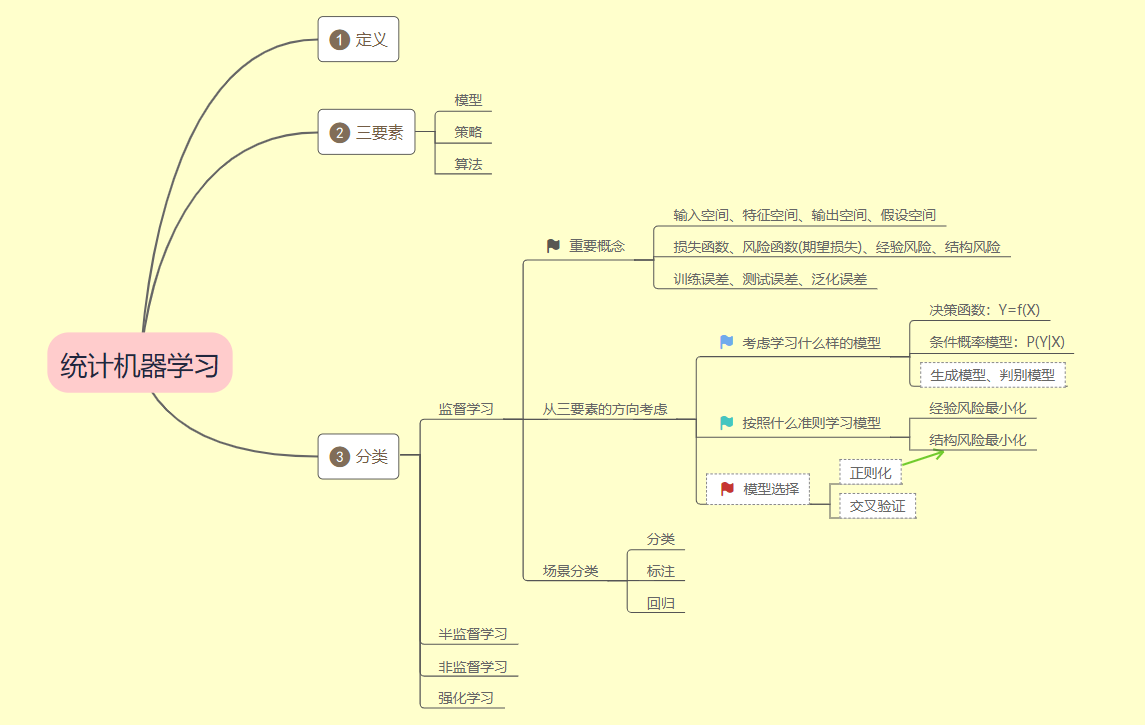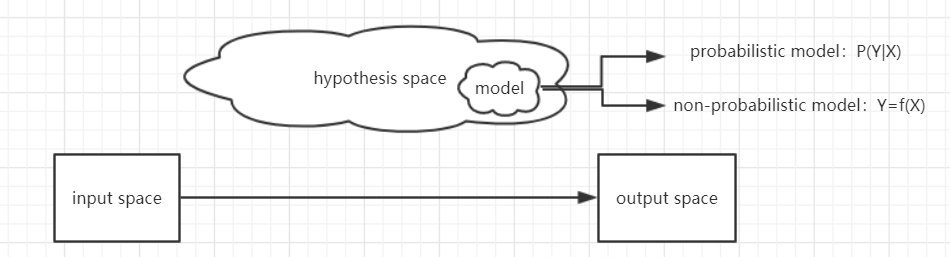### 思维导图### 详细笔记

• 统计学习定义及三要素
• 监督学习中的重要概念、步骤
• 过拟合与模型选择
• 监督学习方法

#### 监督学习中的重要概念

♦ 输入空间(input space)、特征空间(feature space)、输出空间(output space)

♦ 分类(classification)问题、标注(tagging)问题、回归问题

• X和Y均为连续变量的预测问题称为回归问题
回归问题分为学习（函数拟合）和预测两个过程。
评价回归问题就是损失函数了。最常用的损失函数是平方损失函数，由此回归问题可用最小二乘法求解。
• Y为有限个离散变量的预测问题称为分类问题
分类问题分为学习和分类两个过程。
评价分类器性能的指标一般是准确率（accuracy）：对于给定的测试数据集，分类器正确分类的样本数与总样本数之比；
对于二分类问题常用的评价指标是精确率（precision）与召回率（recall），通常以关注的类为正类，其他类为父类；
FN（False Negative）——将正类预测为负类数
FP（False Positive）——将负类预测为正类数
TN（True Negative）——将负类预测为负类数
由此，精确率的定义为：召回率的定义为：还有，$F_1$值，是precision和recall的调和均值：
• X和Y均为变量序列的预测问题称为标注问题
标注问题分为学习和标注两个过程。
评价标注模型的指标与评价分类模型的指标一样。常用统计学方法有HMM、CRF等（后面会一一介绍）

#### 从三要素方面讨论监督学习（以回归问题为例）##### 2. 策略
• 用什么样的准则选择最优的模型.
首先引入损失函数(loss function/cost function)、风险函数(risk function/expected loss)和经验风险(empirical risk/empirical loss)的概念：
Loss function是$f(X)$和$Y$的非负实值函数，记作 度量的是模型一次预测的好坏。
记住几个常用损失函数：
(1) 0-1 loss function: (2) quadratic loss function: (3) absolute loss function: (4) logarithmic/log-likelihood loss function:Expected loss就是loss function关于联合分布的期望。度量的是模型平均意义下预测的好坏。Empirical loss是loss function在训练数据集上的平均。

• 接下来介绍监督学习中的两个基本策略。
♦ 经验风险最小化策略(ERM)，即 其中F是假设空间；
♦ 结构风险最小化(SRM)，即其中J(f)为模型的复杂度，是定义在假设空间$F$上的泛函。
$\lambda\geq0$是系数，用以权衡经验损失和模型复杂度
当样本容量足够大时，经验风险最小化能保证由很好的学习效果；但是，当样本容量很小时，经验风险最小化学习的效果就未必很好，为了防止“过拟合”，提出结构风险最小化。

#### 模型选择

• 正则化
正则化其实就是结构风险最小化的实现，即：

也就是经验风险加上一个正则化项。正则化项一般是模型复杂度的单调递增函数，模型越复杂，正则化值越大。比如正则化项可以是模型参数向量的范数。

• 交叉验证
如果样本充足，可以将其随机分为训练集(training set)、验证集(validation set)、测试集(testing set)。训练集用于训练模型，验证集用来选择模型，测试集用来评估模型。
但是，实际中样本量有限。所以采用交叉验证来重复使用数据：把给定的数据继续切分，将切分的数据集组合为训练集和测试机，在此基础上反复的进行训练、选择和测试。

• Simple cross validation
随机将已知数据分为两部分，一部分作为训练集，另一部分作为测试集；
用训练集在不同参数个数下等训练模型；
在测试集上评估各个模型地测试误差，选出测试误差最小地模型。
• S-fold cross validation
随机将已知数据平均分为$S$个子集；
用$S-1$个子集的数据训练模型，余下的子集测试模型；
对可能的$S$种选择重复进行；
选出$S$次评测中平均误差最小的模型
• Leave-one-out cross validation
用于数据极度缺乏的情况。
$S$=$N$

#### 监督学习方法分类

• 生成方法
由数据学习联合概率分布$P(Y|X)=\frac{P(X,Y)}{P(X)}$,然后求出条件概率分布$P(Y|X)$作为预测的模型。
典型的生成模型有：朴素贝叶斯、HMM；
• 判别方法
由数据直接学习决策函数$f(X)$或者$P(Y|X)$作为预测的函数。
典型的判别模型有：k近邻法、感知机、决策树、逻辑回归、最大熵、SVM、CRF；

### 习题

• 说明伯努利模型的极大似然估计以及贝叶斯估计中的统计学习方法三要素.
A：伯努利模型为条件概率模型，极大似然估计为经典统计学派策略，贝叶斯估计贝叶斯统计学派策略
• 通过经验风险最小化推到极大似然估计，证明模型是条件概率分布，当损失函数是对数损失函数时，经验风险最小化等价于极大似然估计.
A：$R_{emp}=\frac{1}{N}\sum_{i=1}^NL(y_i,f(x_i))=\frac{1}{N}\sum_{i=1}^N-logP(y_i|x_i)$
$=-\frac{1}{N}\sum_{i=1}^NlogP(y_i|x_i)=-\frac{1}{N}\prod_{i=1}^NP(y_i|x_i)$